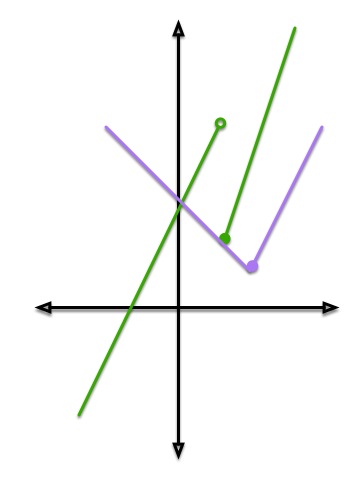# It Might Not Exist

Calculus Level 2

$f(x) = \begin{cases} 3x-1 , \quad \text{ if } x\geq1 \\ 2x+3, \quad \text{ if } x<1 \end{cases}, \quad \quad g(x) = \begin{cases} 3-x ,\; \; \quad \text{ if } x<2 \\ 2x-3, \quad \text{ if } x\geq2 \end{cases}$

Consider the two piecewise functions as shown above. Compute $\displaystyle \lim_{x\to2} f(g(x))$.

Submit -1000 as your answer if you think that the limit fails to exist.×